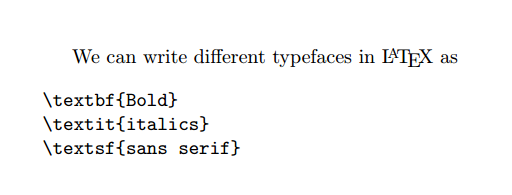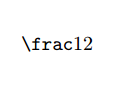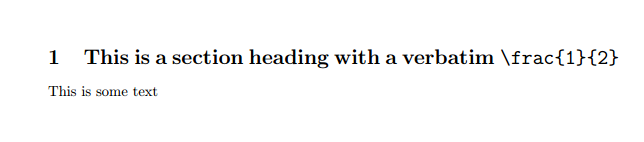Showing first {{hits.length}} results of {{hits_total}} for {{searchQueryText}}{{hits.length}} results for {{searchQueryText}}

This error appears when you have used a verbatim command incorrectly. If you do this, you will generate the error below: main.tex, line 5 LaTeX Error: \verb ended by end of line. See the LaTeX manual or LaTeX Companion for explanation. Type H for immediate help. ... l.7 \unbrace{\verb+\error+} Try typing to proceed. If that doesn't work, type X to quit. To resolve this error, it is best to use the verbatim environment instead as shown below: \begin{verbatim} You should include your verbatim text in here. \end{verbatim} Common Causes Including a line-break inside a \verb command: One common cause for this error to occur is if you have used a \verb command, but you have included a line-break, as shown below: We can write different typefaces in \LaTeX as \verb{ \textbf{Bold} \textit{italics} \textsf{sans serif} } This will generate an error, as line-breaks are not allowed inside the \verb command. The correct way to write this is to use the verbatim environment as shown below: % In your preamble \usepackage{verbatim} % In the main body of your document We can write different typefaces in \LaTeX as \begin{verbatim} \textbf{Bold} \textit{italics} \textsf{sans serif} \end{verbatim}Omitting an end character in a \verb command: The verb command should be used as shown below: \verb!\frac{1}{2}! Note that delimiters here are not unique, and any character will do. The reason that we have used ! as our delimiter here is that there are no ! written in \frac{1}{2}. If we had chosen to write \verb{\frac{1}{2}}, we would generate the output below, which is not correct. \verb{\frac{1}{2}}This can lead to problems, as an error will be generated if the start and end of the \verb are not the same character. Because of this, all of the below commands will generate an error: \verb \frac{1}{2} {\verb \frac{1}{2}} \verb!\frac{1}{2} Once again, it is recommended to simply use the verbatim environment to write this as shown below \begin{verbatim} \frac{1}{2} \end{verbatim} Using a \verb command inside another command: Another way in which this error can appear is if a \verb command is used inside another command as shown below \section{\verb!\frac{1}{2}!} This is not allowed in LaTeX, and is one of the few cases when the verbatim environment won't help. To resolve this error, you should load the cprotect package as shown below: % In your preamble \usepackage{cprotect} % In the main body of your document \cprotect\section{This is a section heading with a verbatim \verb!\frac{1}{2}!} This is some text